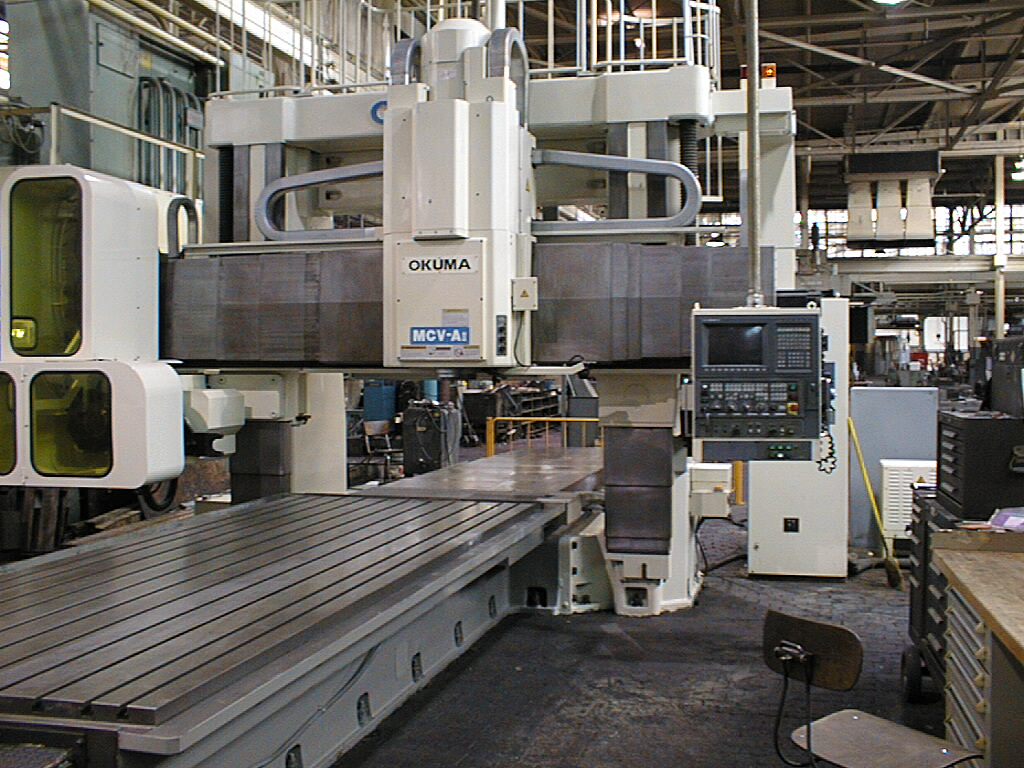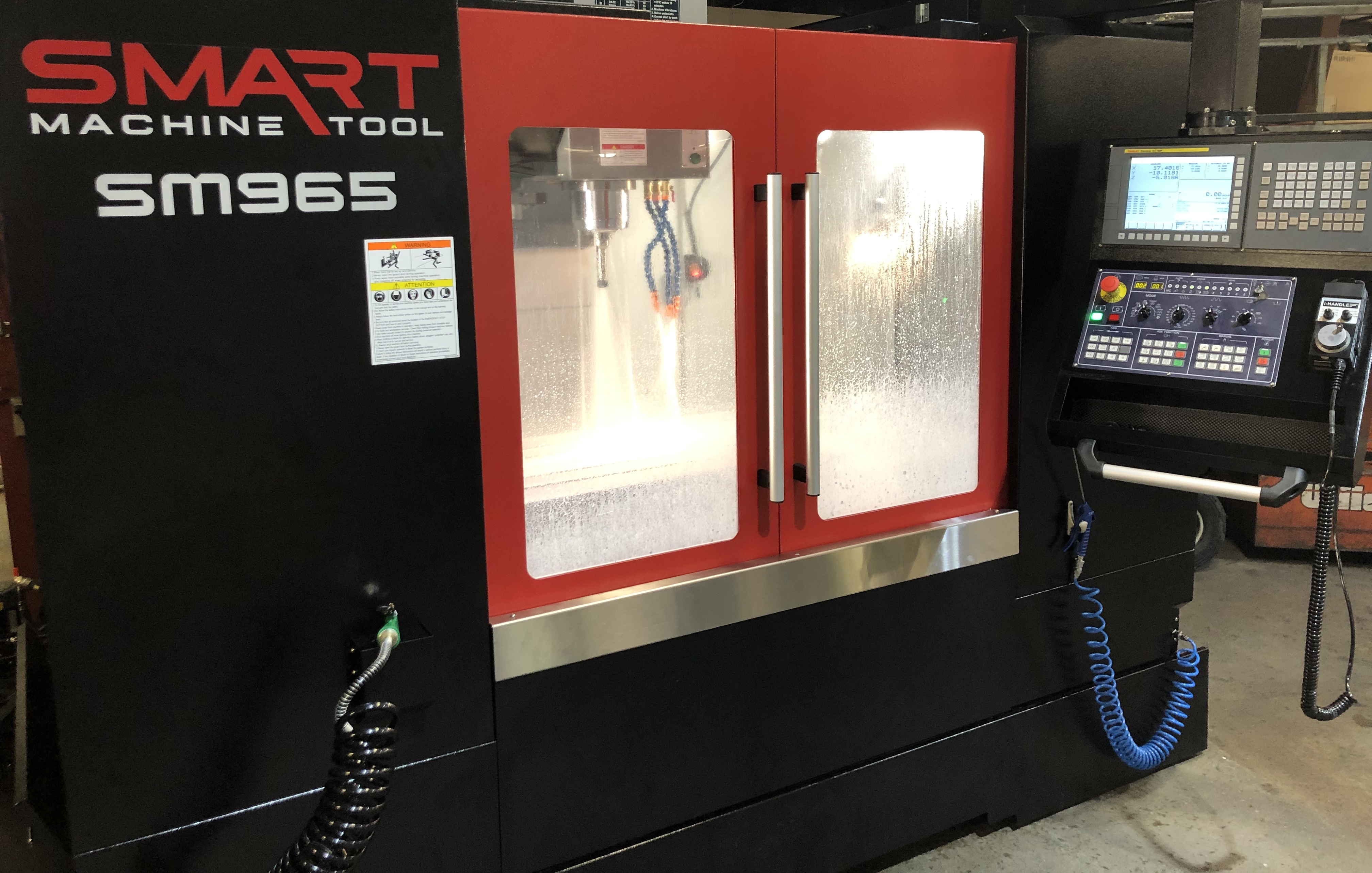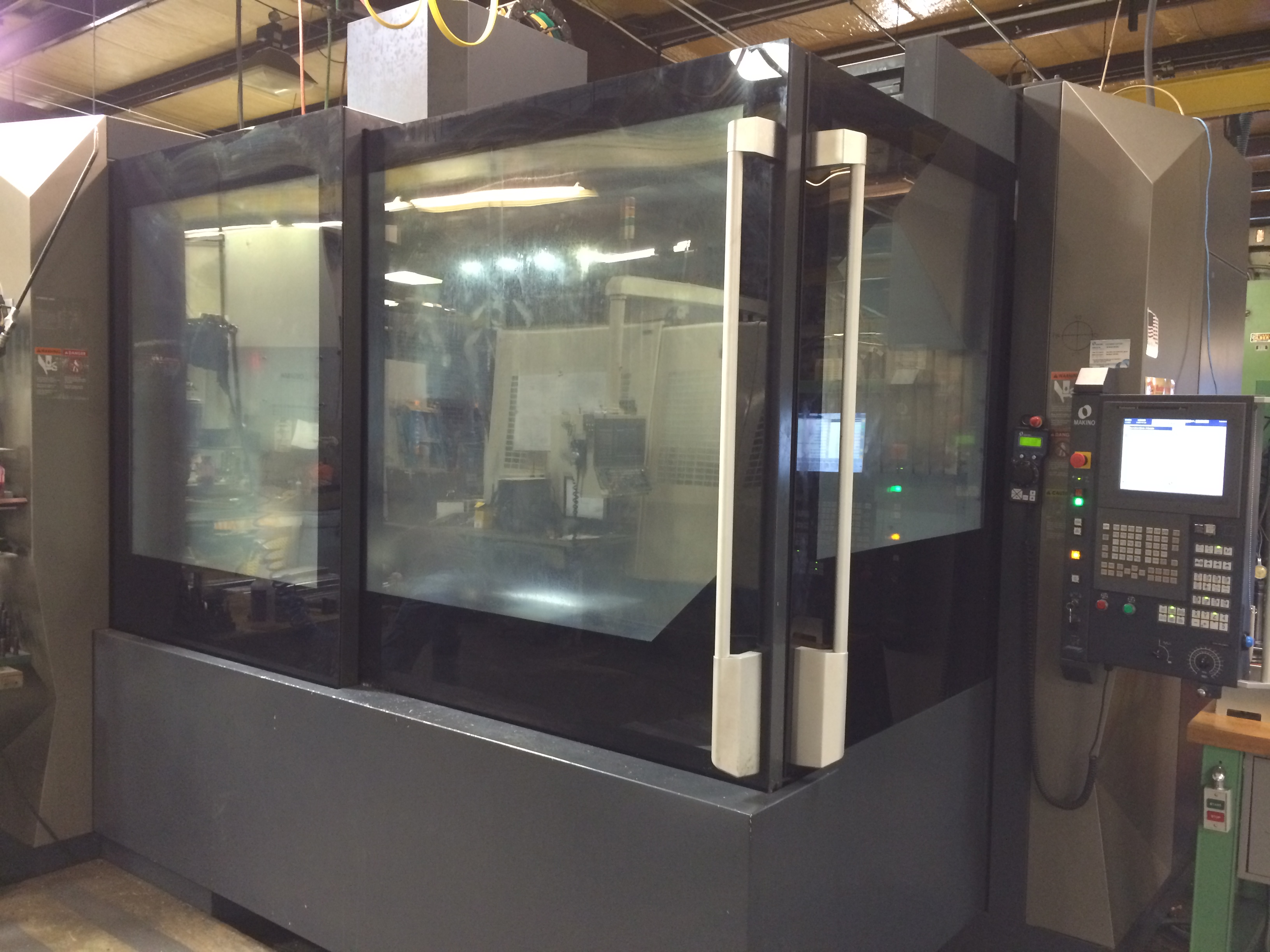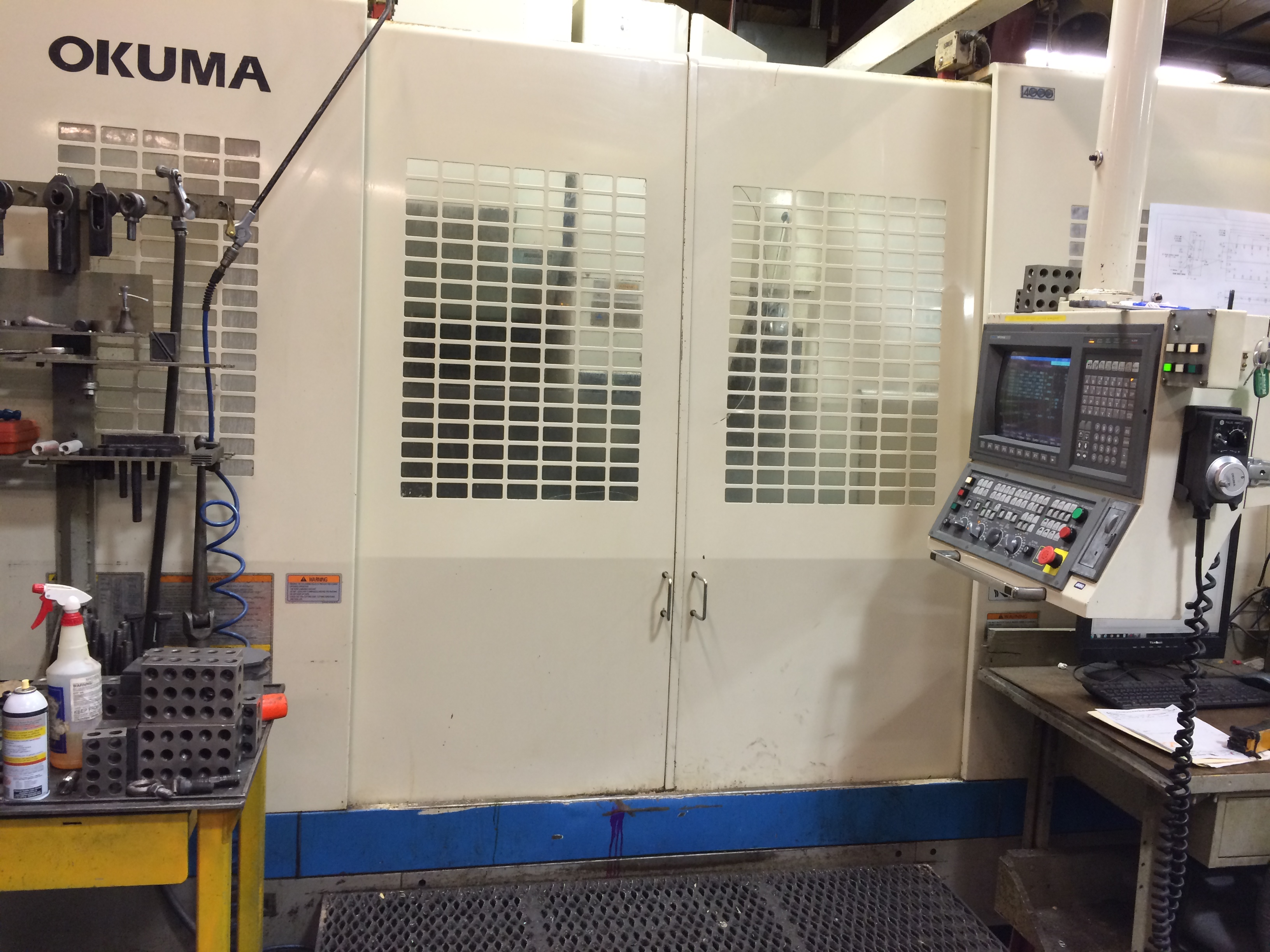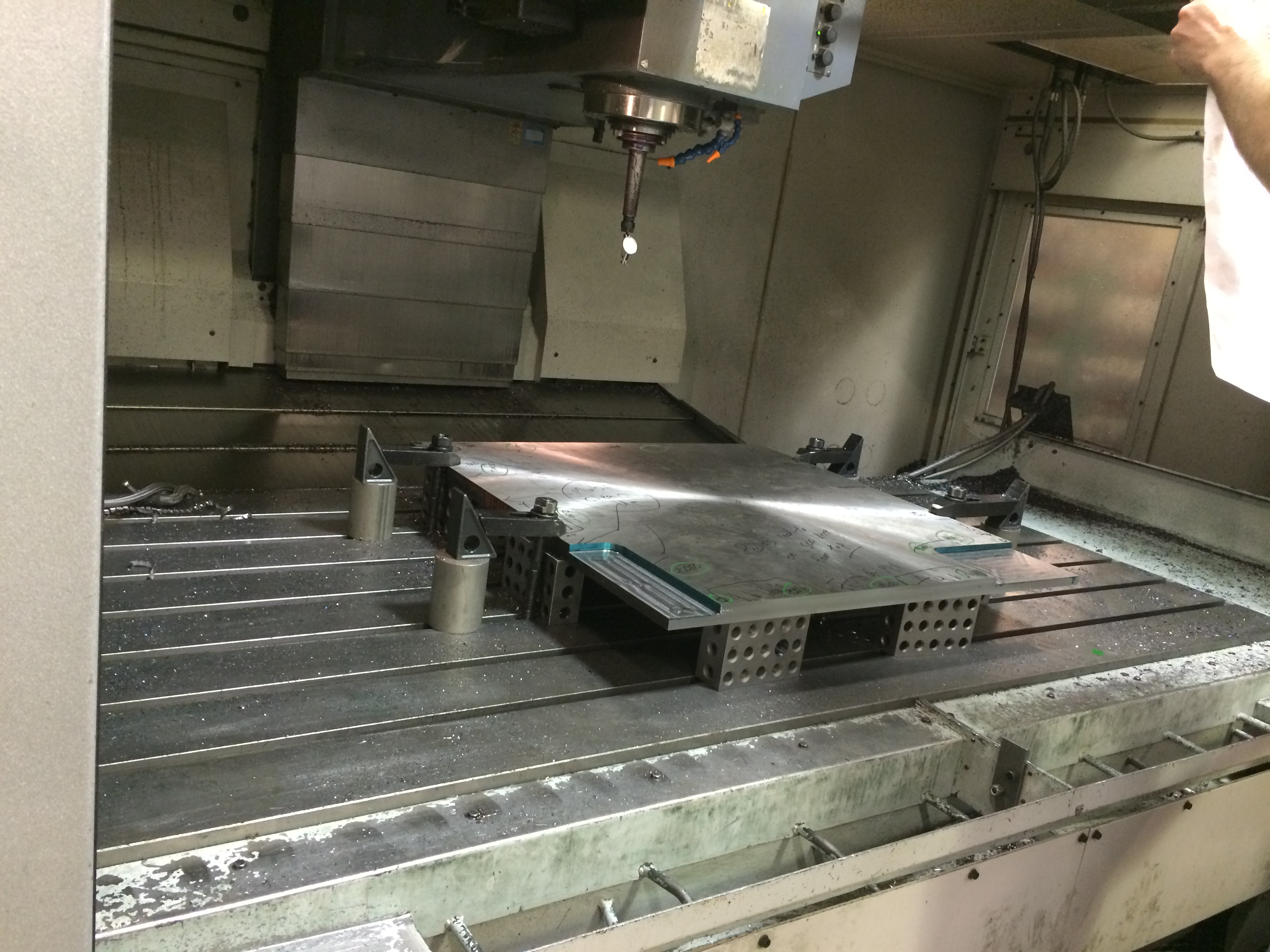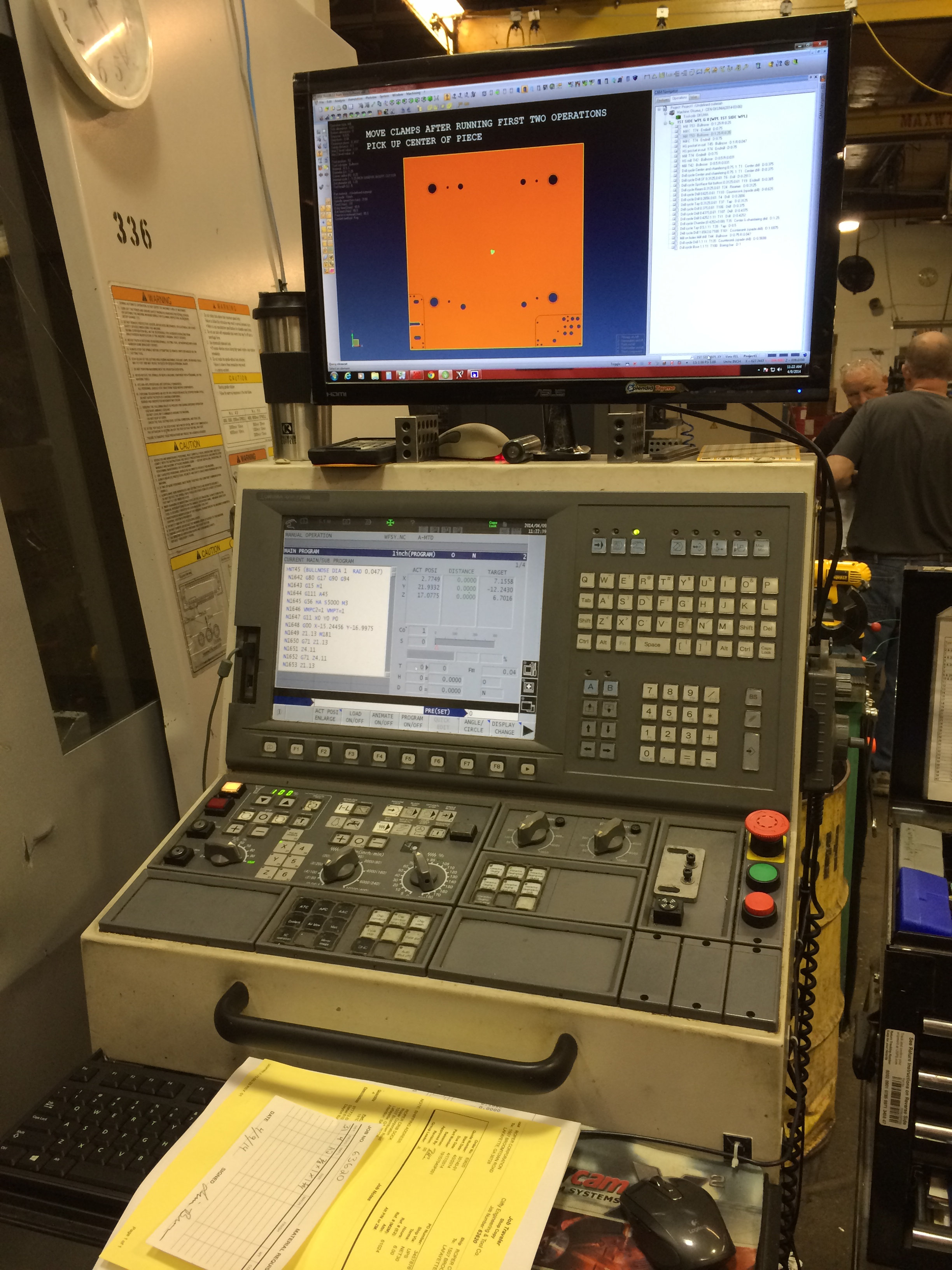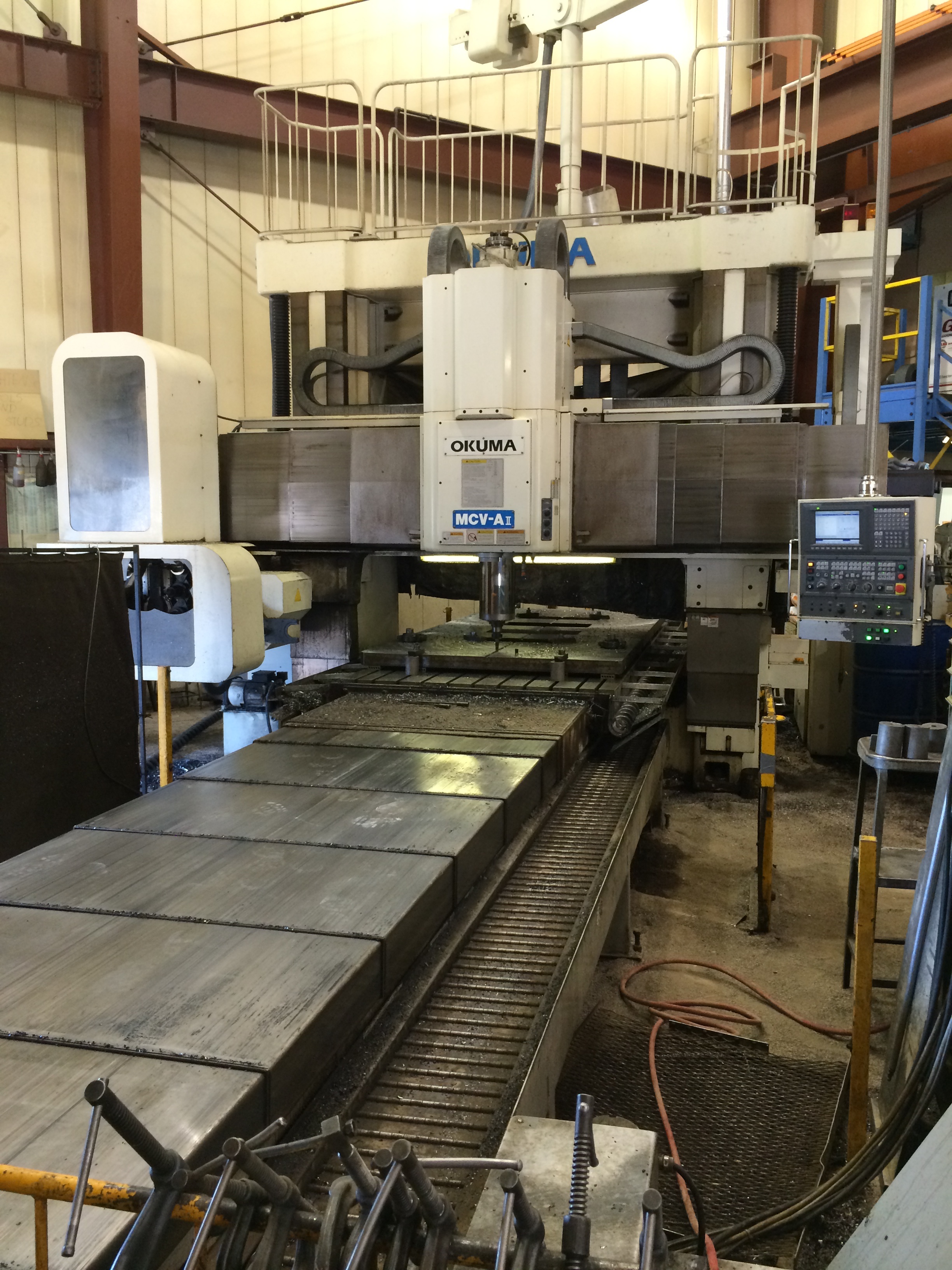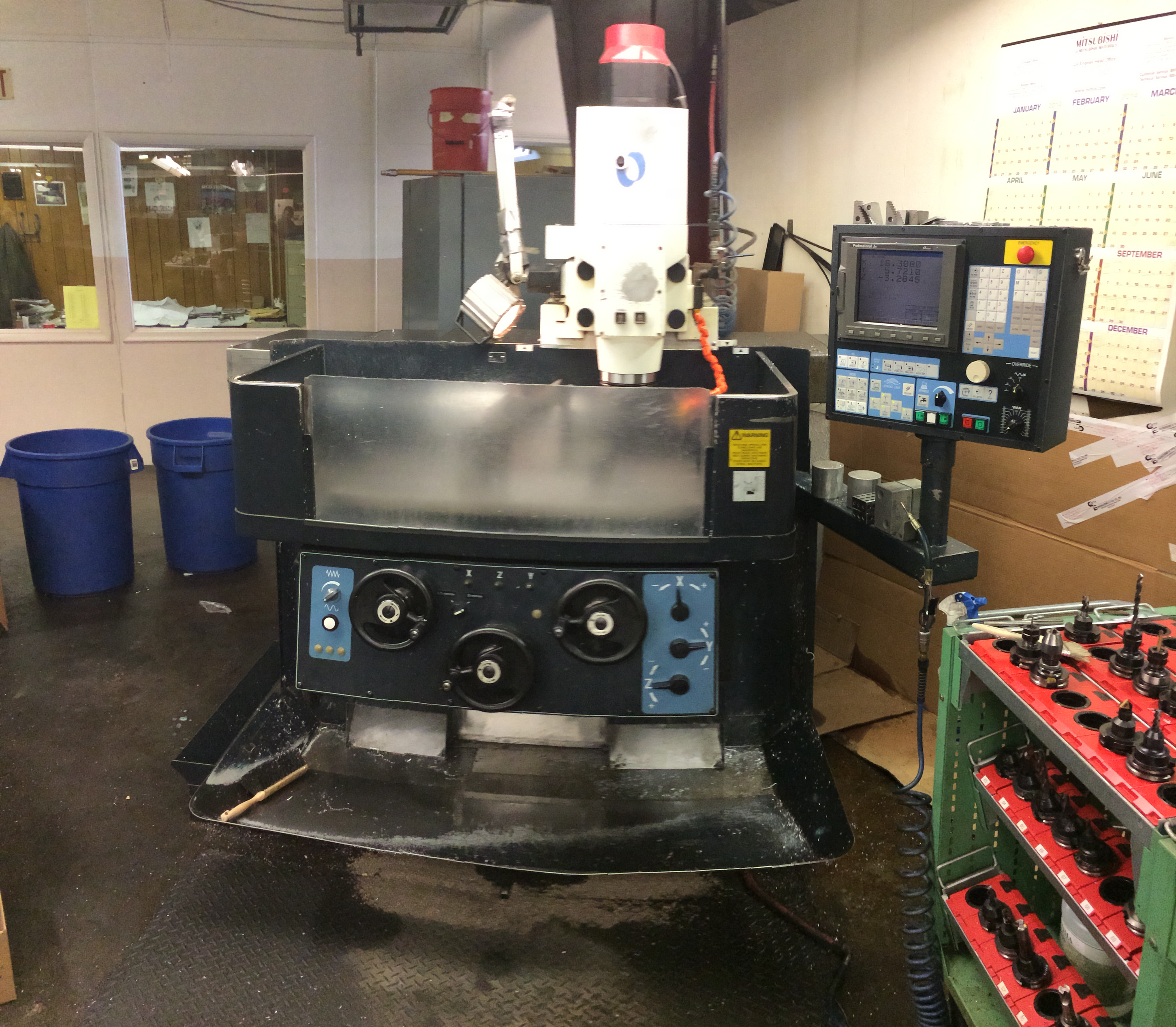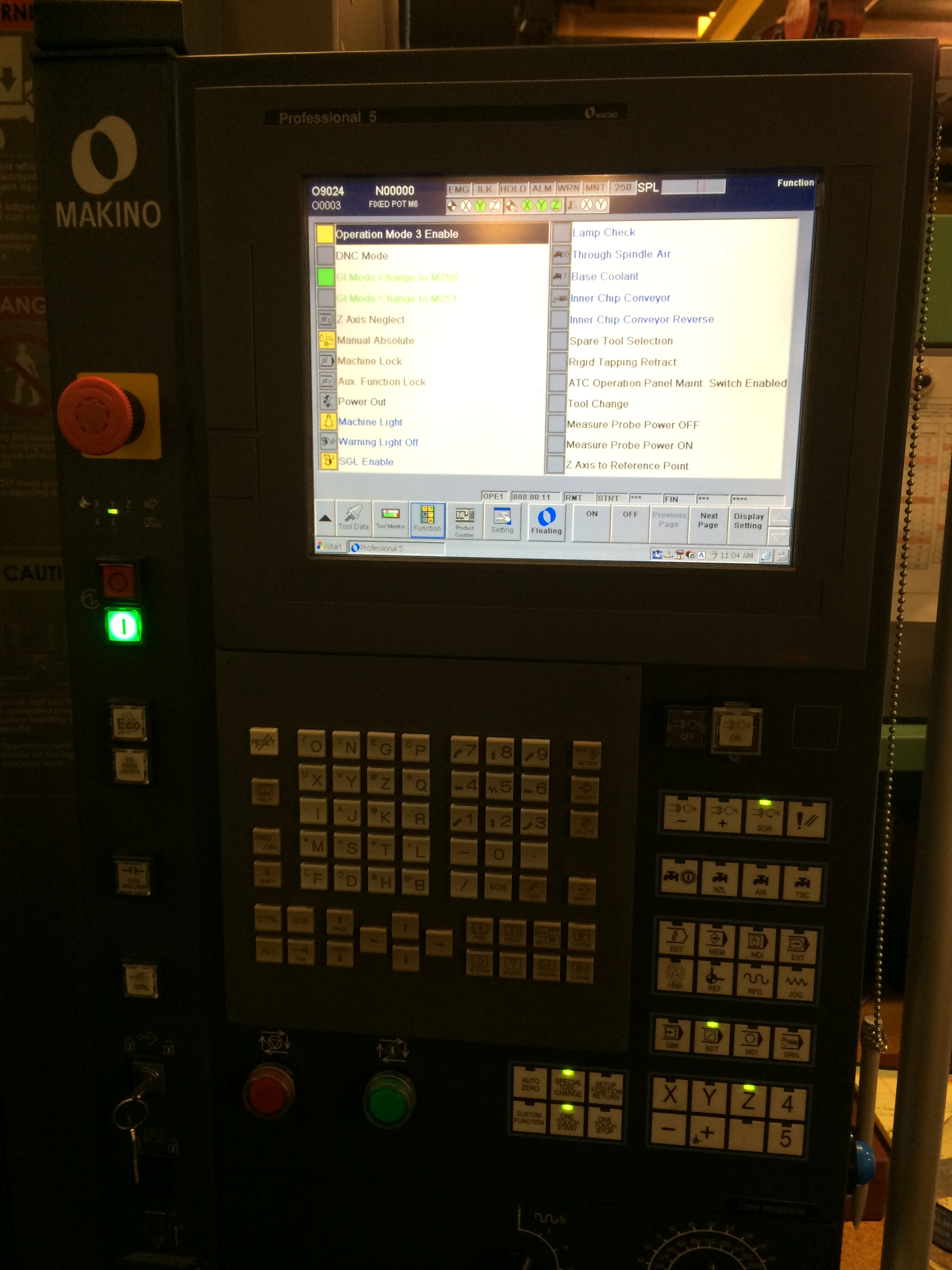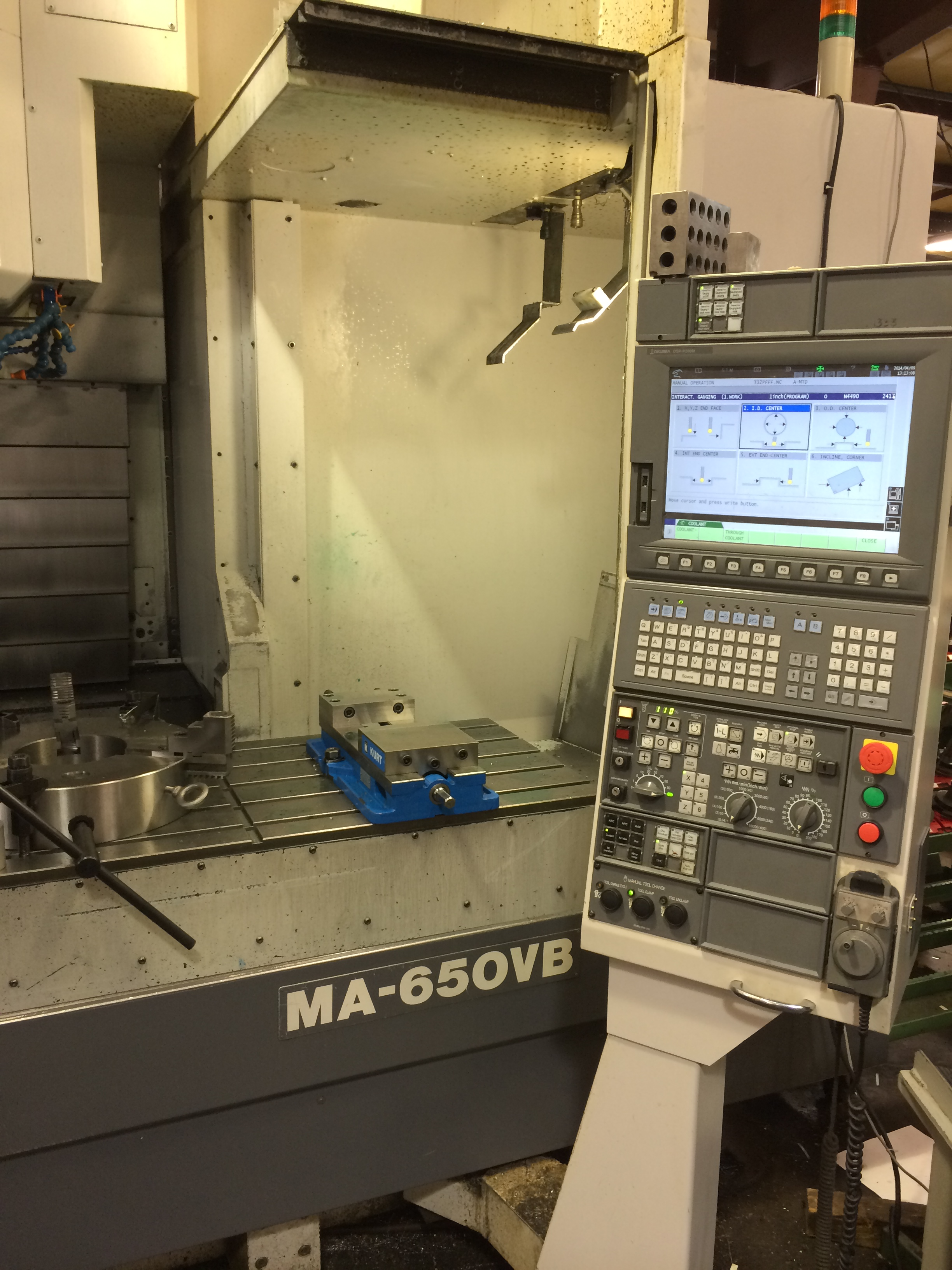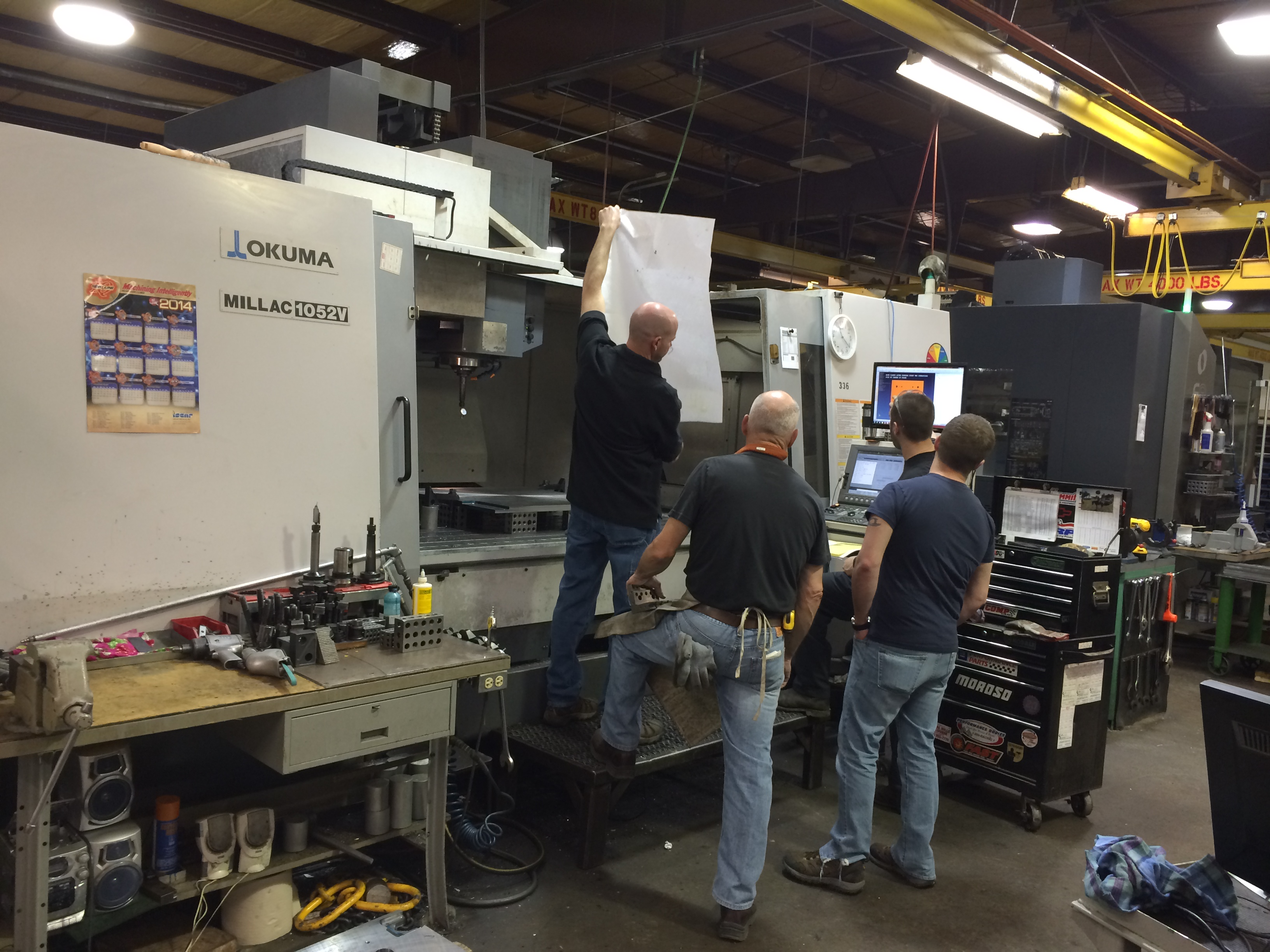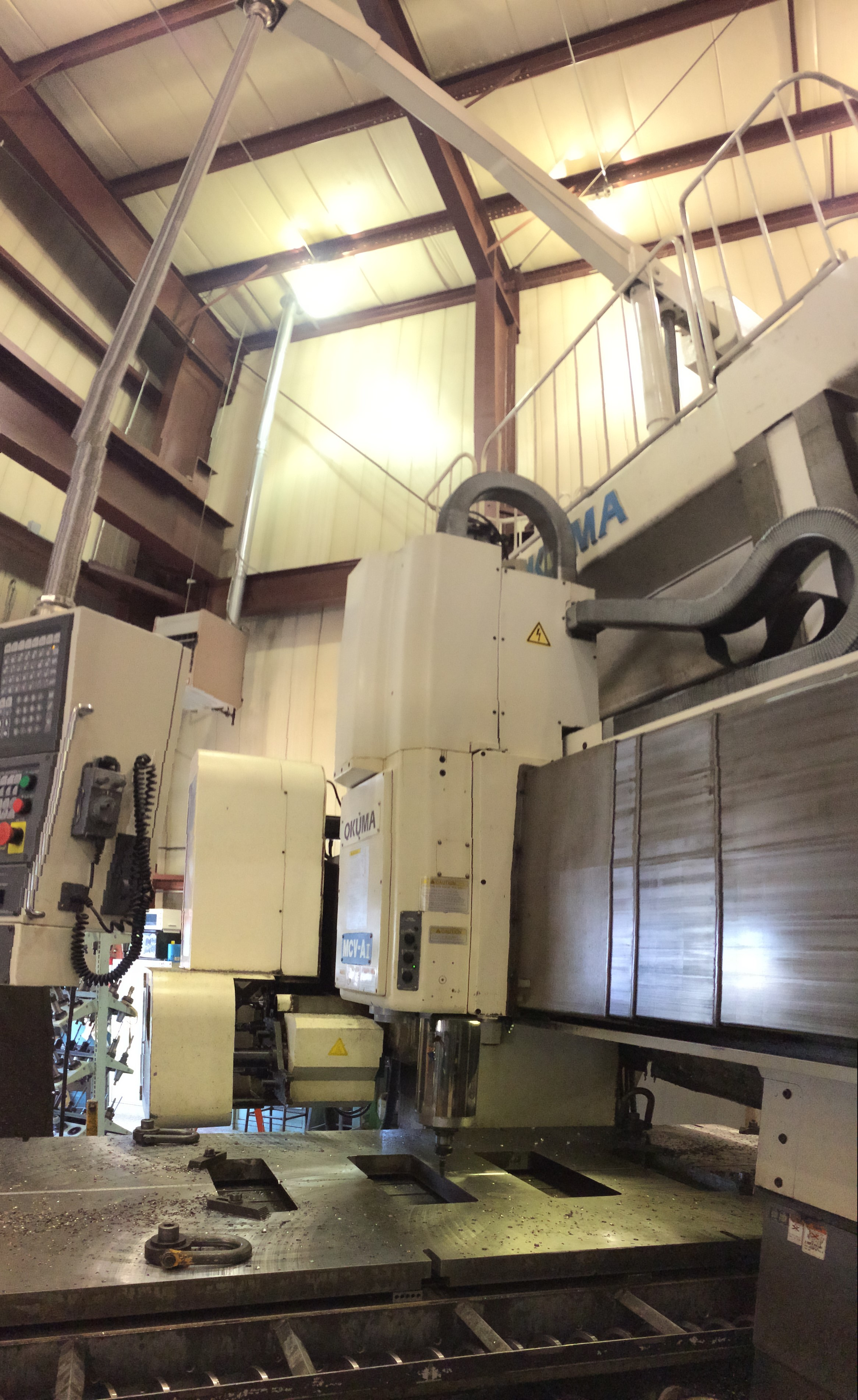## Our CNC MIlling Machines..

• OKUMA MCV-A Double Column VMC - Table Size: 60" x 161" (X= 157.48" Y= 78.74" Z= 52")

• OKUMA GENOS M560V - Table Size: 22.05" x 51.18" (X= 41.34" Y= 22.05" Z= 18.11")

• (2) Smart SM965 VMC - Table Size: 43.31 x 25.59 (X= 35.43" Y= 25.59" Z= 24.02")

• (2) Smart SM1165 VMC - Table Size: 47.24 x 25.29 (X= 43.31" Y= 25.59" Z= 24.02")

• Smart SM1565 - Table Size: 62.99 x 25.59 (X= 59.06" Y= 25.59" Z= 24.02")

• Smart SM1688 - Table Size: 70.87 x 37.40 (X= 62.99" Y= 34.65" Z= 27.56")

• Makino F9 Vertical Machining Center - Table Size: 72.8” x 31.5” (X= 63",Y= 31.5", Z= 25.6")

• Okuma MILAC 1052V VMC - Table Size: 86.6" x 41.34" (X=80.71”, Y=41.73”, Z=31.5)

• Okuma MA-650VB VMC - Table Size: 60.23" x 25.98" (X=60.2”, Y= 25.9”, Z= 24.0)

• (2) EZTrak Bridgeport

• (4) Makino KE55